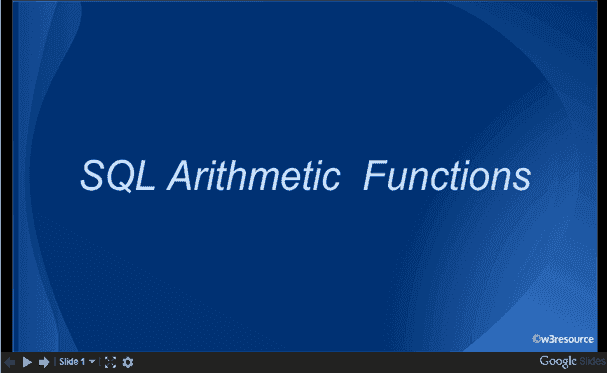# SQL arithmetic function

## Arithmetic function

A mathematical function executes a mathematical operation usually based on input values that are provided as arguments, and return a numeric value as the result of the operation. Mathematical functions operates on numeric data such as decimal, integer, float, real, smallint, and tinyint.

By default, the precision of built-in operations on float data type data is six decimal places.

## SQL arithmetic functions are:

Functions Description
ABS() This SQL ABS() returns the absolute value of a number passed as an argument.
CEIL() This SQL CEIL() will rounded up any positive or negative decimal value within the function upwards.
FLOOR() The SQL FLOOR() rounded up any positive or negative decimal value down to the next least integer value.
EXP() The SQL EXP() returns e raised to the n-th power(n is the numeric expression), where e is the base of natural algorithm and the value of e is approximately 2.71828183.
LN() The SQL LN() function returns the natural logarithm of n, where n is greater than 0 and its base is a number equal to approximately 2.71828183.
MOD() This SQL MOD() function returns the remainder from a division.
POWER() This SQL POWER() function returns the value of a number raised to another, where both of the numbers are passed as arguments.
SQRT() The SQL SQRT() returns the square root of given value in the argument.

Here is a new document which is a collection of questions with short and simple answers, useful for learning SQL as well as for interviews.

Slide presentation:Practice SQL Exercises